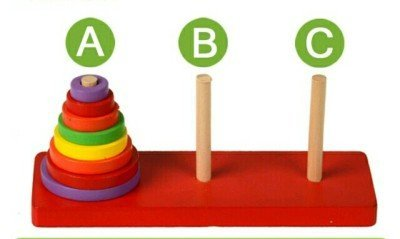### 汉诺塔该怎么玩，方法H⑴ = 1

H(n) = 2×H（n-1）＋1 （n＞1）

H(n) = 2^n – 1 (n>0)

J(n) = 2*H(n) = 2*（2^n – 1） = 2^(n+1）-2